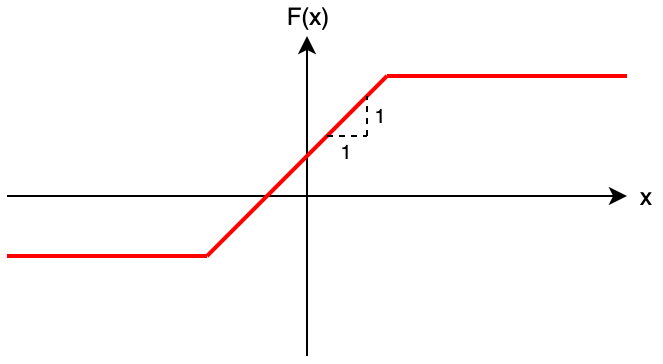Contest Duration: - (local time) (100 minutes) Back to Home

## E - Filters Editorial by en_translator

A $$\Theta(NQ)$$-time solution of applying $$f_1, f_2, \dots, f_N$$ to each of $$x_1, x_2, \dots, x_Q$$ will lead to TLE, because $$NQ$$ can be up to $$4 \times 10^{10}$$.
Now let us consider how the composite function $$F(x) = f_N(\dots f_2(f_1(x))\dots)$$ looks like.

When $$t_i = 1$$, $$f_i$$ is an operation of shifting the graph vertically;
when $$t_i = 2$$, $$f_i$$ is an operation of clamping the graph to at least $$a_i$$;
when $$t_i = 3$$, $$f_i$$ is an operation of clamping the graph to at most $$a_i$$.
Thus, we can expect that the composition of those operations forms like the graph below — namely, there exist numbers $$a, b, c$$ such that $$F(x) = \min(c, \max(b, x + a))$$.We can prove the fact in the following way:

When $$t_i = 1$$, $$f_i(x) = \min(\infty, \max(-\infty, x + a_i))$$;
when $$t_i = 2$$, $$f_i(x) = \min(\infty, \max(a_i, x + 0))$$;
when $$t_i = 3$$, $$f_i(x) = \min(a_i, \max(-\infty, x + 0))$$; so we can always express $$f_i$$ in the form of $$\min(c, \max(b, x + a))$$.

Also, let $$f(x) = \min(c_1, \max(b_1, x + a_1)), g(x) = \min(c_2, \max(b_2, x + a_2))$$; then their composite function $$g(f(x))$$ satisfies

\begin{aligned} g(f(x)) &= \min(c_2, \max(b_2, \min(c_1, \max(b_1, x + a_1)) + a_2))\\ &= \min(c_2, \max(b_2, \min(c_1 + a_2, \max(b_1 + a_2, x + a_1 + a_2))))\\ &= \min(\min(c_2, \max(b_2, c_1 + a_2)), \max(\max(b_2, \min(c_1 + a_2, b_1 + a_2)), x + (a_1 + a_2))),\\ \end{aligned}

so it can be represented as $$\min(c, \max(b, x + a))$$.

Since $$F(x)$$ are the composition of $$f_1, f_2, …, f_N$$, it is proved that be represented in the form of $$\min(c, \max(b, x + a))$$.

Next, let us find $$a, b, c$$ when it is expressed as $$F(x) = \min(c, \max(b, x + a))$$.
These can be calculated by compositing based on the composition rule $$g(f(x)) = \min(\min(c_2, \max(b_2, c_1 + a_2)), \max(\max(b_2, \min(c_1 + a_2, b_1 + a_2)), x + (a_1 + a_2)))$$ described above.

Once we have $$a, b,$$ and $$c$$, we can calculate $$F(x)$$ in $$O(1)$$ time each, so the problem has been solved in a total of $$O(N + Q)$$ time.

Sample Code (C++)

#include <iostream>
#include <numeric>
#include <algorithm>
using namespace std;
using ll = long long;
const ll INF = numeric_limits<ll>::max() / 4;
void chmin(ll& a, ll b){ if(a > b) a = b; }
void chmax(ll& a, ll b){ if(a < b) a = b; }

int main(){
ll N;
cin >> N;
ll low = -INF, high = INF, add = 0;
for(ll i = 0; i < N; i++){
ll A, T;
cin >> A >> T;
if(T == 1){
low += A;
high += A;
}
else if(T == 2){
chmax(low, A);
chmax(high, A);
}
else{
chmin(low, A);
chmin(high, A);
}
}
ll Q;
cin >> Q;
for(ll i = 0; i < Q; i++){
ll x;
cin >> x;
cout << clamp(x + add, low, high) << '\n';
}
}


Sample Code (Python)

N = int(input())
low = -1 << 60
high = 1 << 60
for i in range(N):
A, T = map(int, input().split())
if T == 1:
low += A
high += A
elif T == 2:
if low < A:
low = A
if high < A:
high = A
else:
if low > A:
low = A
if high > A:
high = A
input()
for x in map(int, input().split()):